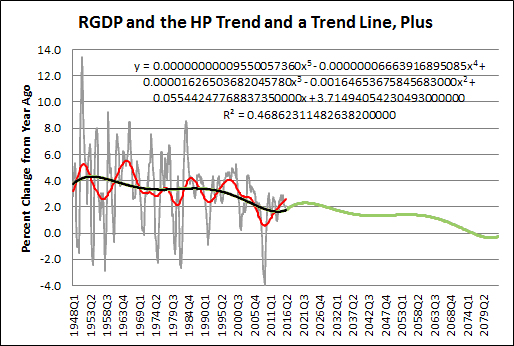## Friday, June 3, 2016

###Imagining the Future

Gray: "percent change from year ago" of Real GDP.
Red: The Hodrick-Prescott of the Gray line.
Black: A fifth order polynomial trend line placed by Excel. Yeah, I don't know what a fifth order polynomial is, either. But it seems to fit the red line pretty well.Graph #1
Imagine the Future: Copy the black line and paste it onto the end of itself. RGDP growth peaks at 2.3% in 2022, and eventually falls below zero. Shown below in green:Graph #2. Click Graph for Larger Image
In case you were wondering: I used Excel's trend line formula to calculate values for the green line. Subtracted 2 from the first calculated value to get the first predicted value and repeated that calc for each subsequent value.

I originally increased the Excel calc to 12 decimal places, but the accuracy was off quite a bit. So I went with 20 decimal places. Now the green line matches the black from 1948 to 2016.

If the RGDP growth trend falls as much in the next 68 years as it has in the last 68, the growth trend will be below zero. To me this says the decline we have had is more troubling than I thought.

// The Excel file